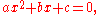xOverview

In mathematics
Mathematics
Mathematics is the study of quantity, space, structure, and change. Mathematicians seek out patterns and formulate new conjectures. Mathematicians resolve the truth or falsity of conjectures by mathematical proofs, which are arguments sufficient to convince other mathematicians of their validity...

, a quadratic equation is a univariate
Univariate
In mathematics, univariate refers to an expression, equation, function or polynomial of only one variable. Objects of any of these types but involving more than one variable may be called multivariate...

polynomial equation of the second degree
Degree of a polynomial
The degree of a polynomial represents the highest degree of a polynominal's terms , should the polynomial be expressed in canonical form . The degree of an individual term is the sum of the exponents acting on the term's variables...

. A general quadratic equation can be written in the formwhere x represents a variable
Variable (mathematics)
In mathematics, a variable is a value that may change within the scope of a given problem or set of operations. In contrast, a constant is a value that remains unchanged, though often unknown or undetermined. The concepts of constants and variables are fundamental to many areas of mathematics and...

or an unknown, and a, b, and c are constant
Constant term
In mathematics, a constant term is a term in an algebraic expression has a value that is constant or cannot change, because it does not contain any modifiable variables. For example, in the quadratic polynomialx^2 + 2x + 3,\ the 3 is a constant term....

s with a ≠ 0. (If a = 0, the equation is a linear equation
Linear equation
A linear equation is an algebraic equation in which each term is either a constant or the product of a constant and a single variable....

.)

The constants a, b, and c are called respectively, the quadratic coefficient
Coefficient
In mathematics, a coefficient is a multiplicative factor in some term of an expression ; it is usually a number, but in any case does not involve any variables of the expression...

, the linear coefficient and the constant term
Constant term
In mathematics, a constant term is a term in an algebraic expression has a value that is constant or cannot change, because it does not contain any modifiable variables. For example, in the quadratic polynomialx^2 + 2x + 3,\ the 3 is a constant term....

or free term.Q;The solution to the quadratic equation x^2-11x+22=0 are x=3 and x=6 .What is the base(radix) of the numbers??Can somebody help me solving this equation x^2 {1-(x/2)}^1/2What does it mean when the discriminant is 0 compared to when it is greater than 0? What does it mean when a, b, and c are integers, and the discrimin...why quadratic equation have only 2 roots? give the proof.when the equation is x2-(m-3)x +m-4 b = how much then if delta greater than zero what is the sign of the quadratic equation ?how to did you get the quadratic formula?DiscussionsAlgabraneed to know how to solve this equation?